Cart
× This is a Sterling guide price. You will be invoiced in the appropriate currency for your country.
• ISBN:9781108461672
• Subject(s):Mathematics
• Qualification:Cambridge AS and A Level
• Author(s):Julia Fletcher, Elaine Dorsett, Colin Nye
• Available from: July 2018

This series has been developed specifically for the Cambridge International AS & A Level Mathematics (9709) syllabus to be examined from 2020.

× This is a Sterling guide price. You will be invoiced in the appropriate currency for your country.

Send a Query

formRow
×
• DESCRIPTION

• Contents
• FEATURES
• Coverage of all five units of Cambridge AS & A Level Mathematics in a single teacher's resource allows for easy lesson planning.

• Example PowerPoint slides help give you ideas on how to present new concepts to an AS & A Level Mathematics classroom.

• Lesson notes contain guidance on the Explore activities within the coursebooks to help you organise your teaching.

• CONTENTS
• Pure Mathematics 1: 1. Quadratics
• 2. Functions
• 3. Coordinate geometry
• 4. Circular measure
• 5. Trigonometry
• 6. Series
• 7. Differentiation
• 8. Further differentiation
• 9. Integration
• Answers. Pure Mathematics 2 & 3: 1. Algebra
• 2. Logarithmic and exponential functions
• 3. Trigonometry
• 4. Differentiation
• 5. Integration
• 6. Numerical solutions of equations
• 7. Further algebra
• 8. Further calculus
• 9. Vectors
• 10. Differential equations
• 11. Complex numbers
• Answers. Mechanics: 1. Velocity and acceleration
• 2. Force and motion in one dimension
• 3. Forces in two dimensions
• 4. Friction
• 5. Connected particles
• 6. General motion in a straight line
• 7. Momentum
• 8. Work and energy
• 9. The work-energy principle and power
• Answers. Probability & Statistics 1: 1. Representation of data
• 2. Measures of central tendency
• 3. Measures of variation
• 4. Probability
• 5. Permutations and combinations
• 6. Probability distributions
• 7. The binomial and geometric distributions
• 8. The normal distribution
• Answers. Probability & Statistics 2: 1. Hypothesis testing
• 2. Poisson distribution
• 3. Linear combinations of random variables
• 4. Continuous random variables
• 5. Sampling
• 6. Estimation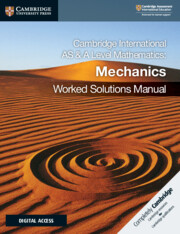\$22.80 Mechanics Worked Solutions Manual with Digital Access (2 Years) View product\$26.45 Pure Mathematics 1 Worked Solutions Manual with Digital Access View product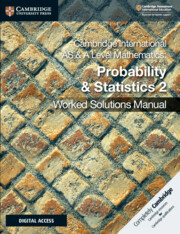\$22.80 Probability & Statistics 2 Worked Solutions Manual with Digital Access View product\$22.80 Probability & Statistics 1 Worked Solutions Manual with Digital Access View product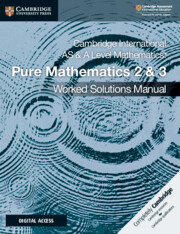\$26.45 Pure Mathematics 2 & 3 Worked Solutions Manual with Digital Access View product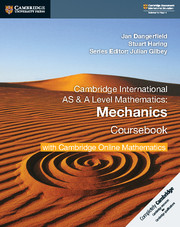\$39.50 Mechanics Coursebook with Cambridge Online Mathematics (2 Years) View product\$39.50 Probability & Statistics 1 Coursebook with Cambridge Online Mathematics (2 Years) View product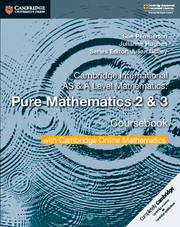\$50.35 Pure Mathematics 2 and 3 Coursebook with Cambridge Online Mathematics (2 Years) View product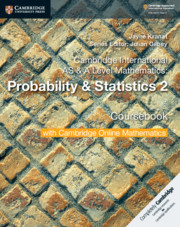\$39.50 Probability & Statistics 2 Coursebook with Cambridge Online Mathematics (2 Years) View product\$50.35 Pure Mathematics 1 Coursebook with Cambridge Online Mathematics (2 Years) View product

Need a local sales consultant?